# Class 10 science ncert solutions chapter 1

## Chemical Reactions And Equations

### Page No 6:

#### Question 1:

Why should a magnesium ribbon be cleaned before it is burnt in air?

Magnesium is an extremely reactive metal. When stored, it reacts with oxygen to form a layer of magnesium oxide on its surface. This layer of magnesium oxide is quite stable and prevents further reaction of magnesium with oxygen. The magnesium ribbon is cleaned by sand paper for removing this layer so that the underlying metal can be exposed to air.

##### Video Solution for chemical reactions and equations (Page: 6 , Q.No.: 1)

NCERT Solution for Class 10 science – chemical reactions and equations 6 , Question 1

#### Question 2:

Write the balanced equation for the following chemical reactions.

(i) Hydrogen + Chlorine → Hydrogen chloride

(ii) Barium chloride + Aluminium sulphate → Barium sulphate +

Aluminium chloride

(iii) Sodium + Water → Sodium hydroxide + Hydrogen

(i)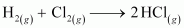(ii)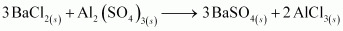(iii)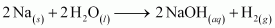##### Video Solution for chemical reactions and equations (Page: 6 , Q.No.: 2)

NCERT Solution for Class 10 science – chemical reactions and equations 6 , Question 2

#### Question 3:

Write a balanced chemical equation with state symbols for the following reactions.

(i) Solutions of barium chloride and sodium sulphate in water react to give insoluble barium sulphate and the solution of sodium chloride.

(ii) Sodium hydroxide solution (in water) reacts with hydrochloric acid solution (in water) to produce sodium chloride solution and water.

(i)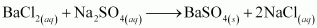(ii)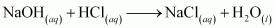##### Video Solution for chemical reactions and equations (Page: 6 , Q.No.: 3)

NCERT Solution for Class 10 science – chemical reactions and equations 6 , Question 3

#### Question 1:

A solution of a substance ‘X’ is used for white washing.

(i) Name the substance ‘X’ and write its formula.

(ii) Write the reaction of the substance ‘X’ named in (i) above with water.

(i) The substance ‘X’ is calcium oxide. Its chemical formula is CaO.

(ii) Calcium oxide reacts vigorously with water to form calcium hydroxide (slaked lime).

##### Video Solution for chemical reactions and equations (Page: 10 , Q.No.: 1)

NCERT Solution for Class 10 science – chemical reactions and equations 10 , Question 1

#### Question 2:

Why is the amount of gas collected in one of the test tubes in Activity 1.7 double of the amount collected in the other? Name this gas.

Water (H2O) contains two parts hydrogen and one part oxygen. Therefore, the amount of hydrogen and oxygen produced during electrolysis of water is in a 2:1 ratio. During electrolysis, since hydrogen goes to one test tube and oxygen goes to another, the amount of gas collected in one of the test tubes is double of the amount collected in the other.

##### Video Solution for chemical reactions and equations (Page: 10 , Q.No.: 2)

NCERT Solution for Class 10 science – chemical reactions and equations 10 , Question 2

#### Question 1:

Why does the colour of copper sulphate solution change when an iron nail is dipped in it?

When an iron nail is placed in a copper sulphate solution, iron displaces copper from copper sulphate solution forming iron sulphate, which is green in colour.

Therefore, the blue colour of copper sulphate solution fades and green colour appears.

##### Video Solution for chemical reactions and equations (Page: 13 , Q.No.: 1)

NCERT Solution for Class 10 science – chemical reactions and equations 13 , Question 1

#### Question 2:

Give an example of a double displacement reaction other than the one given in Activity 1.10.

Sodium carbonate reacts with calcium chloride to form calcium carbonate and sodium chloride.

In this reaction, sodium carbonate and calcium chloride exchange ions to form two new compounds. Hence, it is a double displacement reaction.

##### Video Solution for chemical reactions and equations (Page: 13 , Q.No.: 2)

NCERT Solution for Class 10 science – chemical reactions and equations 13 , Question 2

#### Question 3:

Identify the substances that are oxidised and the substances that are reduced in the following reactions.

(i)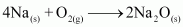(ii)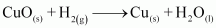(i) Sodium (Na) is oxidised as it gains oxygen and oxygen gets reduced.

(ii) Copper oxide (CuO) is reduced to copper (Cu) while hydrogen (H2) gets oxidised to water (H2O).

##### Video Solution for chemical reactions and equations (Page: 13 , Q.No.: 3)

NCERT Solution for Class 10 science – chemical reactions and equations 13 , Question 3

#### Question 1:

Which of the statements about the reaction below are incorrect?

(b) Carbon dioxide is getting oxidised.

(c) Carbon is getting oxidised.

(d) Lead oxide is getting reduced.

(i) (a) and (b)

(ii) (a) and (c)

(iii) (a), (b) and (c)

(iv) all

(i)(a) and (b)

##### Video Solution for chemical reactions and equations (Page: 14 , Q.No.: 1)

NCERT Solution for Class 10 science – chemical reactions and equations 14 , Question 1

#### Question 2:

The above reaction is an example of a

(a) combination reaction.

(b) double displacement reaction.

(c) decomposition reaction.

(d) displacement reaction.

(d) The given reaction is an example of a displacement reaction.

#### Question 3:

What happens when dilute hydrochloric acid is added to iron filings? Tick the correct answer.

(a) Hydrogen gas and iron chloride are produced.

(b) Chlorine gas and iron hydroxide are produced.

(c) No reaction takes place.

(d) Iron salt and water are produced.

(a) Hydrogen gas and iron chloride are produced. The reaction is as follows:

##### Video Solution for chemical reactions and equations (Page: 15 , Q.No.: 3)

NCERT Solution for Class 10 science – chemical reactions and equations 15 , Question 3

#### Question 4:

What is a balanced chemical equation? Why should chemical equations be balanced?

A reaction which has an equal number of atoms of all the elements on both sides of the chemical equation is called a balanced chemical equation.

The law of conservation of mass states that mass can neither be created nor destroyed. Hence, in a chemical reaction, the total mass of reactants should be equal to the total mass of the products. It means that the total number of atoms of each element should be equal on both sides of a chemical equation. Hence, it is for this reason that chemical equations should be balanced.

##### Video Solution for chemical reactions and equations (Page: 15 , Q.No.: 4)

NCERT Solution for Class 10 science – chemical reactions and equations 15 , Question 4

#### Question 5:

Translate the following statements into chemical equations and then balance them.

(a) Hydrogen gas combines with nitrogen to form ammonia.

(b) Hydrogen sulphide gas burns in air to give water and sulphur dioxide.

(c) Barium chloride reacts with aluminium sulphate to give aluminium chloride and a precipitate of barium sulphate.

(d) Potassium metal reacts with water to give potassium hydroxide and hydrogen gas.

(a)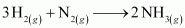(b)(c)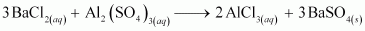(d)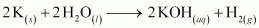##### Video Solution for chemical reactions and equations (Page: 15 , Q.No.: 5)

NCERT Solution for Class 10 science – chemical reactions and equations 15 , Question 5

#### Question 6:

Balance the following chemical equations.

(a)

(b)

(c)

(d)

(a)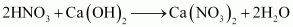(b)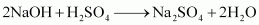(c)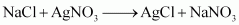(d)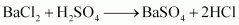##### Video Solution for chemical reactions and equations (Page: 15 , Q.No.: 6)

NCERT Solution for Class 10 science – chemical reactions and equations 15 , Question 6

#### Question 7:

Write the balanced chemical equations for the following reactions.

(a) Calcium hydroxide + Carbon dioxide → Calcium carbonate + Water

(b) Zinc + Silver nitrate → Zinc nitrate + Silver

(c) Aluminium + Copper chloride → Aluminium chloride + Copper

(d) Barium chloride + Potassium sulphate → Barium sulphate +

Potassium chloride

(a)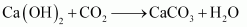(b)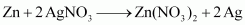(c)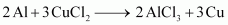(d)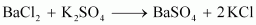##### Video Solution for chemical reactions and equations (Page: 15 , Q.No.: 7)

NCERT Solution for Class 10 science – chemical reactions and equations 15 , Question 7

#### Question 8:

Write the balanced chemical equation for the following and identify the type of reaction in each case.

(a)Potassium bromide (aq) + Barium iodide (aq)­ → Potassium iodide (aq) +

Barium bromide(s)

(b) Zinc carbonate (s) → Zinc oxide (s) + Carbon dioxide (g)

(c) Hydrogen (g) + Chlorine (g) → Hydrogen chloride (g)

(d) Magnesium (s) + Hydrochloric acid (aq) → Magnesium chloride (aq) + Hydrogen (g)

(a)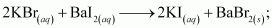Double displacement reaction

(b)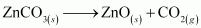; Decomposition reaction

(c)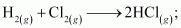Combination reaction

(d)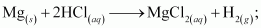Displacement reaction

##### Video Solution for chemical reactions and equations (Page: 15 , Q.No.: 8)

NCERT Solution for Class 10 science – chemical reactions and equations 15 , Question 8

#### Question 9:

What does one mean by exothermic and endothermic reactions? Give examples.

Chemical reactions that release energy in the form of heat, light, or sound are called exothermic reactions.

Example: Mixture of sodium and chlorine to yield table salt

In other words, combination reactions are exothermic.

Reactions that absorb energy or require energy in order to proceed are called endothermic reactions.

For example: In the process of photosynthesis, plants use the energy from the sun to convert carbon dioxide and water to glucose and oxygen.

##### Video Solution for chemical reactions and equations (Page: 15 , Q.No.: 9)

NCERT Solution for Class 10 science – chemical reactions and equations 15 , Question 9

#### Question 10:

Why is respiration considered an exothermic reaction? Explain.

Energy is required to support life. Energy in our body is obtained from the food we eat. During digestion, large molecules of food are broken down into simpler substances such as glucose. Glucose combines with oxygen in the cells and provides energy. The special name of this combustion reaction is respiration. Since energy is released in the whole process, it is an exothermic process.

##### Video Solution for chemical reactions and equations (Page: 15 , Q.No.: 10)

NCERT Solution for Class 10 science – chemical reactions and equations 15 , Question 10

#### Question 11:

Why are decomposition reactions called the opposite of combination reactions? Write equations for these reactions.

Decomposition reactions are those in which a compound breaks down to form two or more substances. These reactions require a source of energy to proceed. Thus, they are the exact opposite of combination reactions in which two or more substances combine to give a new substance with the release of energy.

Decomposition reaction: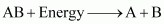Combination reaction: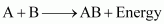##### Video Solution for chemical reactions and equations (Page: 15 , Q.No.: 11)

NCERT Solution for Class 10 science – chemical reactions and equations 15 , Question 11

#### Question 12:

Write one equation each for decomposition reactions where energy is supplied in the form of heat, light or electricity.

(a) Thermal decomposition:

(b) Decomposition by light:

(c) Decomposition by electricity:

##### Video Solution for chemical reactions and equations (Page: 16 , Q.No.: 12)

NCERT Solution for Class 10 science – chemical reactions and equations 16 , Question 12

#### Question 13:

What is the difference between displacement and double displacement reactions? Write equations for these reactions.

In a displacement reaction, a more reactive element replaces a less reactive element from a compound.where A is more reactive than B

In a double displacement reaction, two atoms or a group of atoms switch places to form new compounds.

For example:

Displacement reaction:

Double displacement reaction:

#### Question 14:

In the refining of silver, the recovery of silver from silver nitrate solution involved displacement by copper metal. Write down the reaction involved.

##### Video Solution for chemical reactions and equations (Page: 16 , Q.No.: 14)

NCERT Solution for Class 10 science – chemical reactions and equations 16 , Question 14

#### Question 15:

What do you mean by a precipitation reaction? Explain by giving examples.

A reaction in which an insoluble solid (called precipitate) is formed is called a precipitation reaction.

For example:

In this reaction, calcium carbonate is obtained as a precipitate. Hence, it is a precipitation reaction.

Another example of precipitation reaction is:

In this reaction, barium sulphate is obtained as a precipitate.

##### Video Solution for chemical reactions and equations (Page: 16 , Q.No.: 15)

NCERT Solution for Class 10 science – chemical reactions and equations 16 , Question 15

#### Question 16:

Explain the following in terms of gain or loss of oxygen with two examples each.

(a) Oxidation

(b) Reduction

(a) Oxidation is the gain of oxygen.

For example:

(i)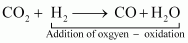(ii)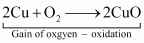In equation (i), H2 is oxidized to H2O and in equation (ii), Cu is oxidised to CuO.

(b) Reduction is the loss of oxygen.

For example:

(i)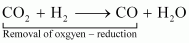(ii)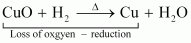In equation (i), CO2 is reduced to CO and in equation (ii), CuO is reduced to Cu.

##### Video Solution for chemical reactions and equations (Page: 16 , Q.No.: 16)

NCERT Solution for Class 10 science – chemical reactions and equations 16 , Question 16

#### Question 17:

A shiny brown-coloured element ‘X’ on heating in air becomes black in colour. Name the element ‘X’ and the black coloured compound formed.

‘X’ is copper (Cu) and the black-coloured compound formed is copper oxide (CuO). The equation of the reaction involved on heating copper is given below.

##### Video Solution for chemical reactions and equations (Page: 16 , Q.No.: 17)

NCERT Solution for Class 10 science – chemical reactions and equations 16 , Question 17

#### Question 18:

Why do we apply paint on iron articles?

Iron articles are painted because it prevents them from rusting. When painted, the contact of iron articles from moisture and air is cut off. Hence, rusting is prevented. So presence of air and moisture is essential for rusting to take place.

##### Video Solution for chemical reactions and equations (Page: 16 , Q.No.: 18)

NCERT Solution for Class 10 science – chemical reactions and equations 16 , Question 18

#### Question 19:

Oil and fat containing food items are flushed with nitrogen. Why?

Nitrogen is an inert gas and does not easily react with these substances. On the other hand, oxygen reacts with food substances and makes them rancid. Thus, bags used in packing food items are flushed with nitrogen gas to remove oxygen inside the pack. When oxygen is not present inside the pack, rancidity of oil and fat containing food items is avoided.

##### Video Solution for chemical reactions and equations (Page: 16 , Q.No.: 19)

NCERT Solution for Class 10 science – chemical reactions and equations 16 , Question 19

#### Question 20:

Explain the following terms with one example each.

(a) Corrosion

(b) Rancidity

(a) Corrosion:

Corrosion is defined as a process where materials, usually metals, deteriorate as a result of a chemical reaction with air, moisture, chemicals, etc.

For example, iron, in the presence of moisture, reacts with oxygen to form hydrated iron oxide.

This hydrated iron oxide is rust.

(b) Rancidity:

The process of oxidation of fats and oils that can be easily noticed by the change in taste and smell is known as rancidity.

For example, the taste and smell of butter changes when kept for long.

Rancidity can be avoided by:

1. Storing food in air tight containers

2. Storing food in refrigerators